# the market supply curve and exit and entry Aplia Homework 6. The market supply curve and exit and entry Aa Aa Cons...

the market supply curve and exit and entry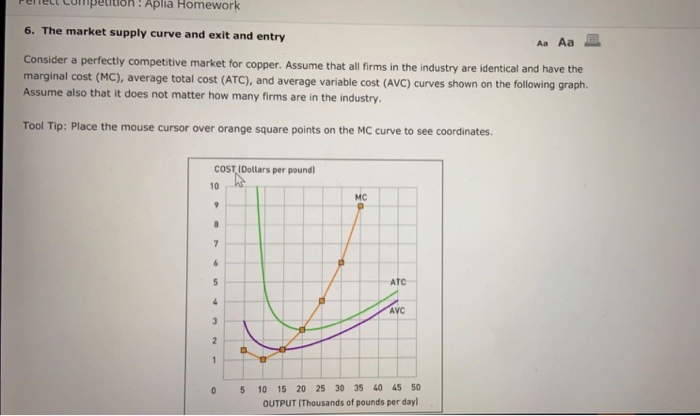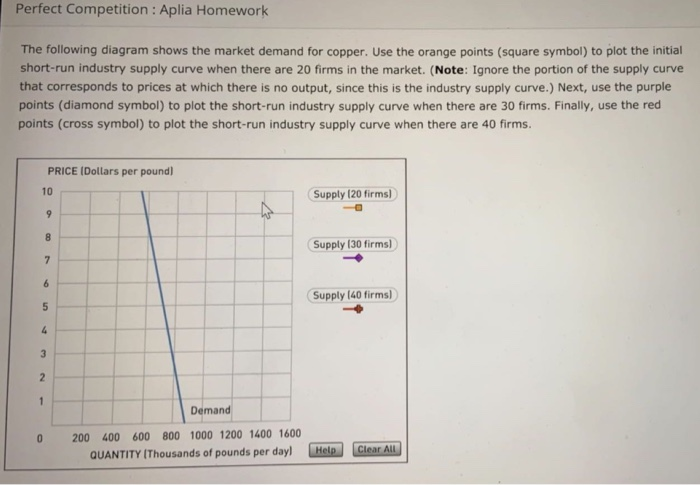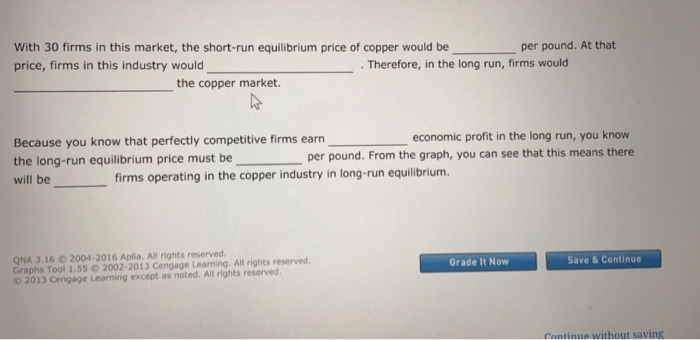Aplia Homework 6. The market supply curve and exit and entry Aa Aa Consider a perfectly competitive market for copper. Assume that all firms in the industry are identical and have the marginal cost (MC), average total cost (ATC), and average variable cost (AVC) curves shown on the following graph. Assume also that it does not matter how many firms are in the industry. Tool Tip: Place the mouse cursor over orange square points on the MC curve to see coordinates. COST IDollars per poundl 10 MC 9 7 6 5 ATC 4 AVC 5 10 15 20 25 30 35 40 45 50 0 OUTPUT IThousands of pounds per dayl
Perfect Competition : Aplia Homework The following diagram shows the market demand for copper. Use the orange points (square symbol) to plot the initial short-run industry supply curve when there are 20 firms in the market. (Note: Ignore the portion of the supply curve that corresponds to prices at which there is no output, since this is the industry supply curve.) Next, use the purple points (diamond symbol) to plot the short-run industry supply curve when there are 30 firms. Finally, use the red points (cross symbol) to plot the short-run industry supply curve when there are 40 firms. PRICE (Dollars per pound 10 Supply (20 firms) 9 8 Supply (30 firms) 7 6 Supply (40 firms) 5 3 2 1 Demand 200 400 600 800 1000 1200 1400 1600 Clear All Help QUANTITY IThousands of pounds per day)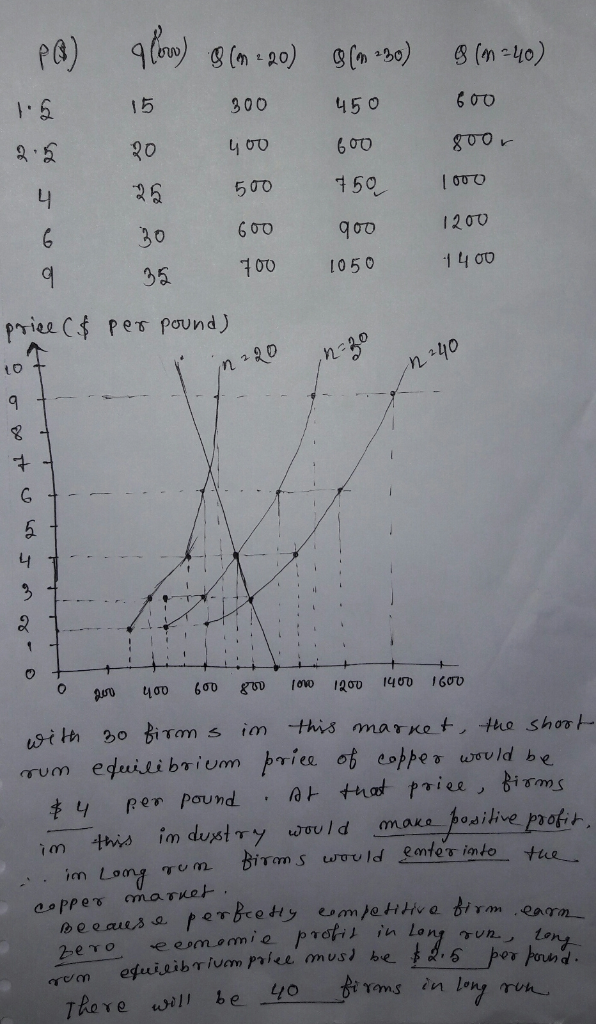##### Add Answer of: the market supply curve and exit and entry Aplia Homework 6. The market supply curve and exit and entry Aa Aa Cons...
Similar Homework Help Questions
• ### 71:06 supply and long-run equillbrium i Consider a perfectly competitive market for titanium. Ass...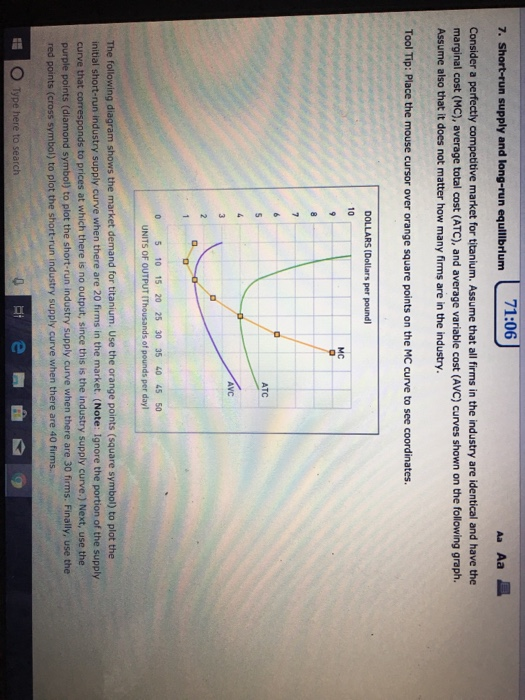super positive i did this wrong. please help. 71:06 supply and long-run equillbrium i Consider a perfectly competitive market for titanium. Assume that all firms in the industry are identical and have the marginal cost (MC), average total cost (ATC), and average variable cost (AVC) curves shown on the following graph. Assume also that it does not matter how many firms are in the industry Tool Tip: Place the mouse cursor over orange square points on the MC curve to...

• ### 6. Short-run supply and long-run equilibrium Consider the competitive market for copper. Assume that, regardless of...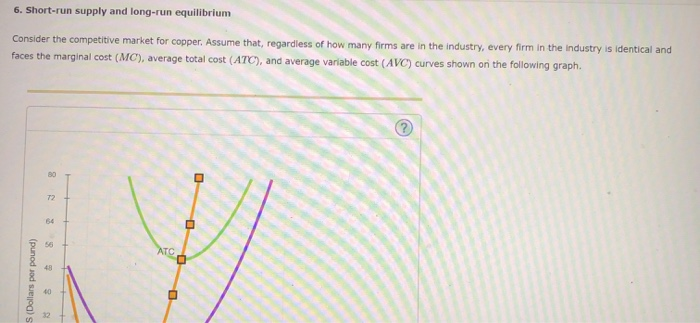6. Short-run supply and long-run equilibrium Consider the competitive market for copper. Assume that, regardless of how many firms are in the industry, every firm in the industry is identical and faces the marginal cost (MC), average total cost (ATC), and average variable cost (AVC) curves shown on the following graph. (punod soda) COSTS (Dollars per pound) AVC 0 + 0 + 3 MC + + 6 9 12 15 18 21 24 QUANTITY (Thousands of pounds) 27 30 -...

• ### 7. Short-run supply and long-run equilibrium Consider the competitive market for copper. Assume that, regardless of...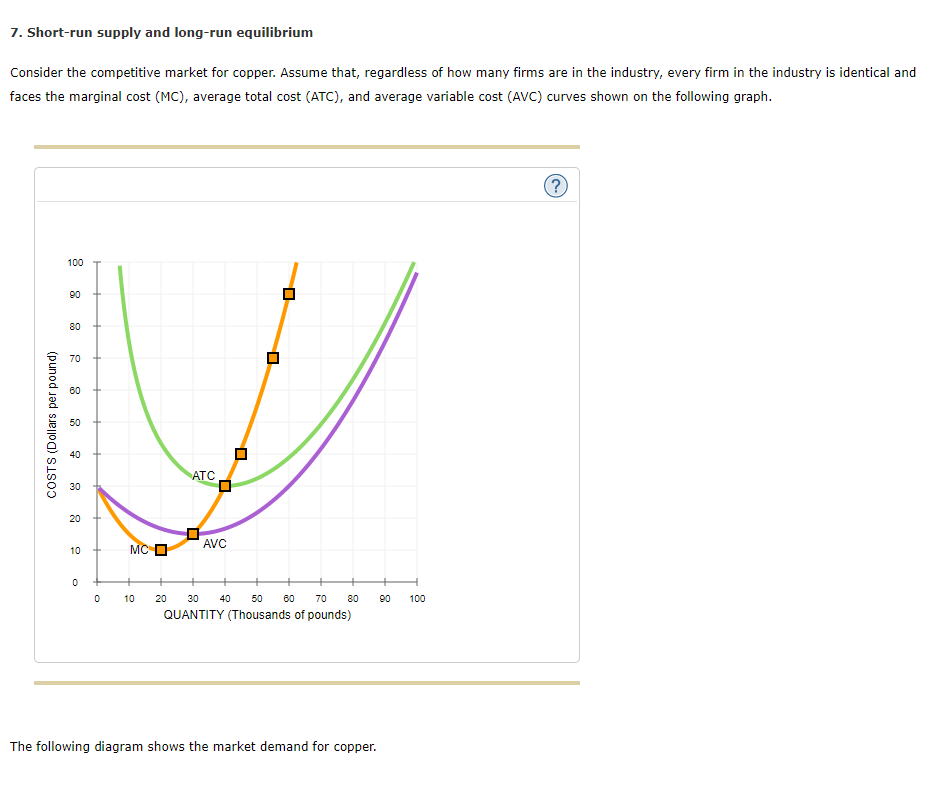7. Short-run supply and long-run equilibrium Consider the competitive market for copper. Assume that, regardless of how many firms are in the industry, every firm in the industry is identical and faces the marginal cost (MC), average total cost (ATC), and average variable cost (AVC) curves shown on the following graph. COSTS (Dollars per pound) MC D AVC 0 + 0 + 10 + + + + + + + 20 30 40 50 60 70 80 QUANTITY (Thousands of...

• ### 7. Short-run supply and long-run equilibrium Consider the competitive market for copper. Assume t...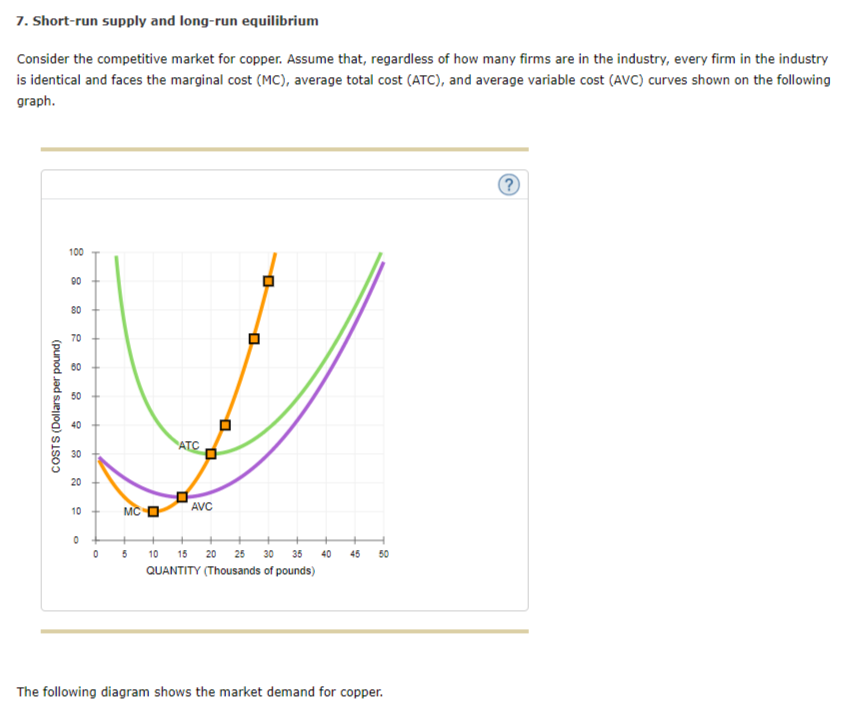7. Short-run supply and long-run equilibrium Consider the competitive market for copper. Assume that, regardless of how many firms are in the industry every finn/n tne industry is identical and faces the marginal cost (MC), average total cost (ATC), and average variable cost (AVC) curves shown on the following graph. 100 90 80 70 60 50 40 30 20 AVC 10 0 5 10 15 20 25 30 35 40 4550 QUANTITY (Thousands of pounds) The following diagram shows the...

• ### 5. Short-run supply and long-run equilibrium Consider the competitive market for copper. Assume that, regardless of...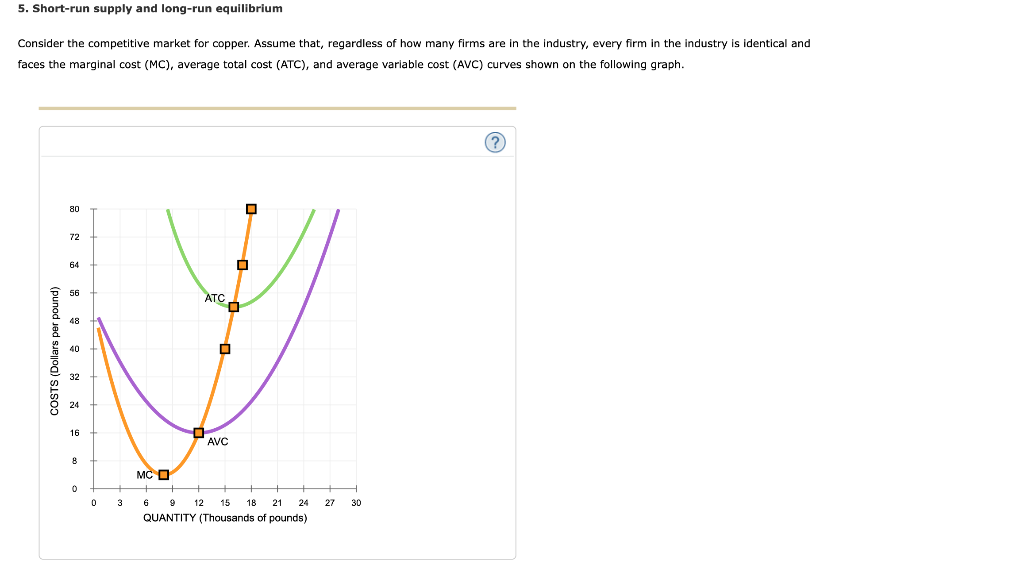5. Short-run supply and long-run equilibrium Consider the competitive market for copper. Assume that, regardless of how many firms are in the industry, every firm in the industry is identical and faces the marginal cost (MC), average total cost (ATC), and average variable cost (AVC) curves shown on the following graph. COSTS (Dollars per pound) AVC MC O 0 3 27 30 6 9 12 15 18 21 24 QUANTITY (Thousands of pounds) The following diagram shows the market demand...

• ### Consider the competitive market for copper. Assume that, regardless of how many firms are in the...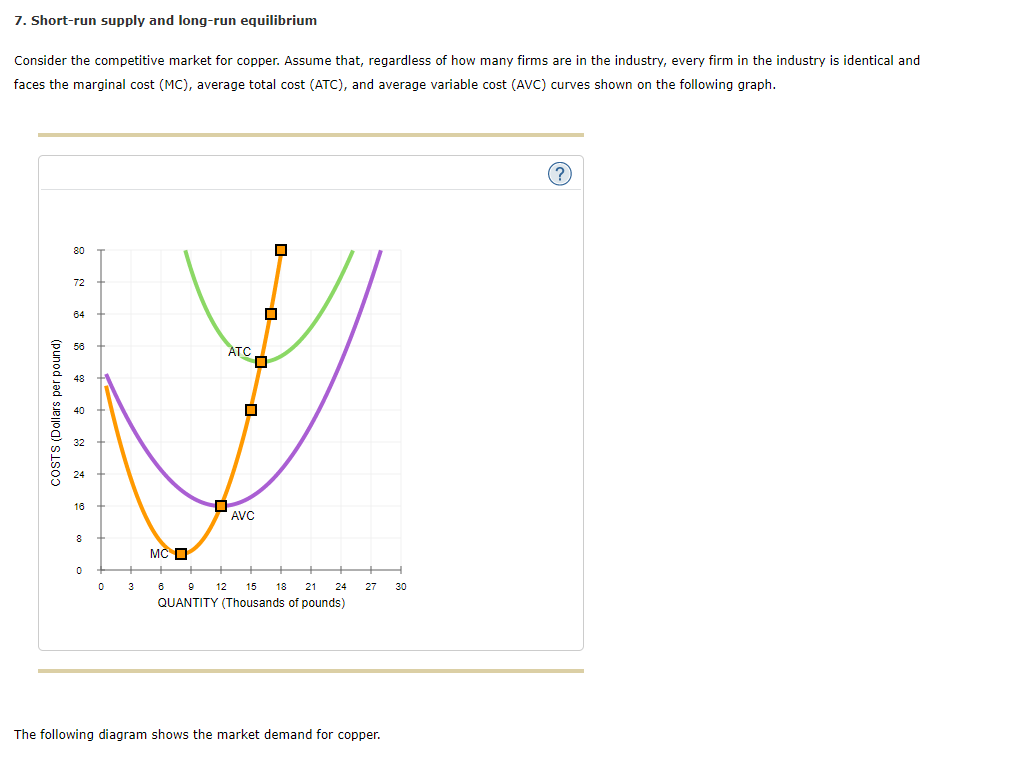Consider the competitive market for copper. Assume that, regardless of how many firms are in the industry, every firm in the industry is identical and faces the marginal cost (MC), average total cost (ATC), and average variable cost (AVC) curves shown on the following graph. 0 3 6 9 12 15 18 21 24 27 30 80 72 64 56 48 40 32 24 16 8 0 COSTS (Dollars per pound) QUANTITY (Thousands of pounds) MC ATC AVC The following...

• ### 8. Short-run supply and long-run equilibrium Consider the perfectly competitive market for copper. Assume that, regardless...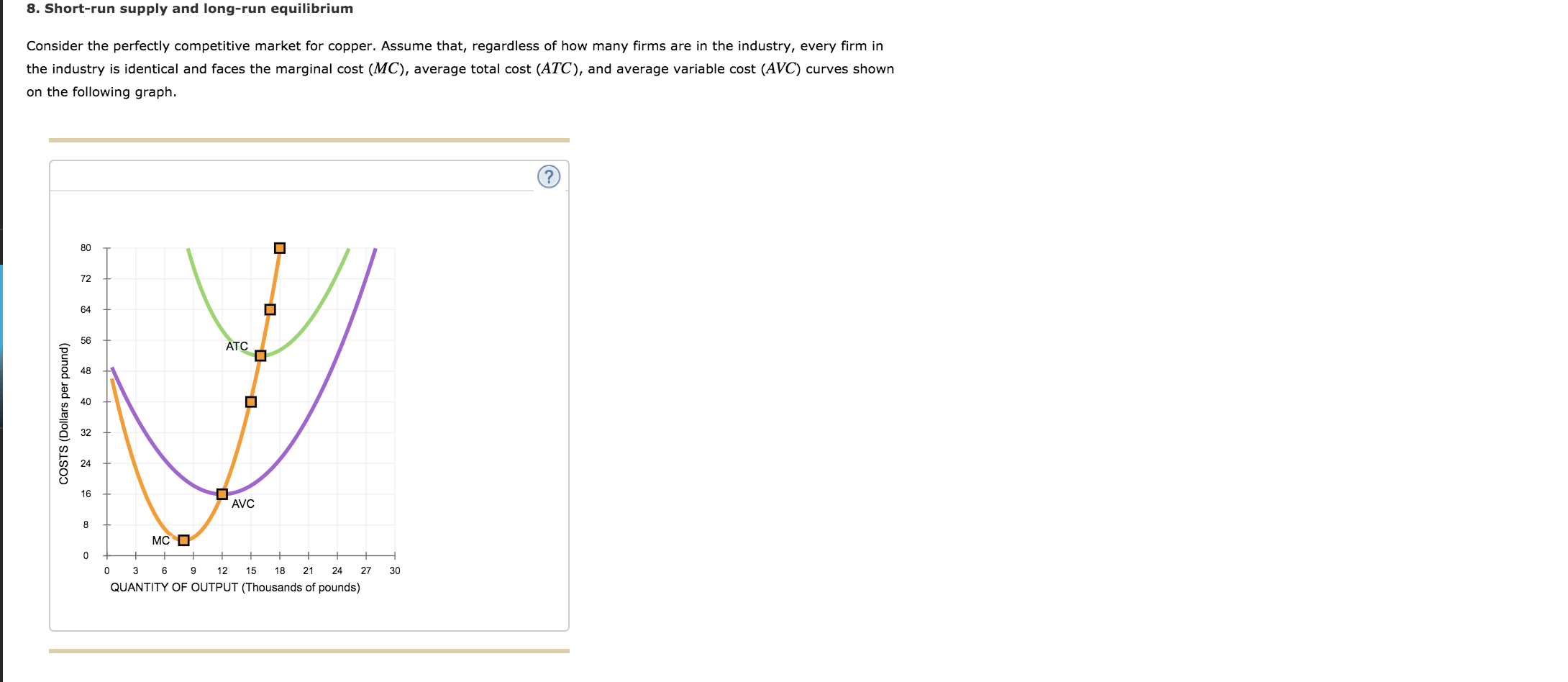8. Short-run supply and long-run equilibrium Consider the perfectly competitive market for copper. Assume that, regardless of how many firms are in the industry, every firm in the industry is identical and faces the marginal cost (MC), average total cost (ATC), and average variable cost (AVC) curves shown on the following graph. ATC COSTS (Dollars per pound) AVC MC D 0 Ft 0 3 6 9 12 15 18 21 24 27 QUANTITY OF OUTPUT (Thousands of pounds) 30 The...

• ### 5. Short-run supply and long-run equilibrium Consider the competitive market for titanium. Assume that, regardless of...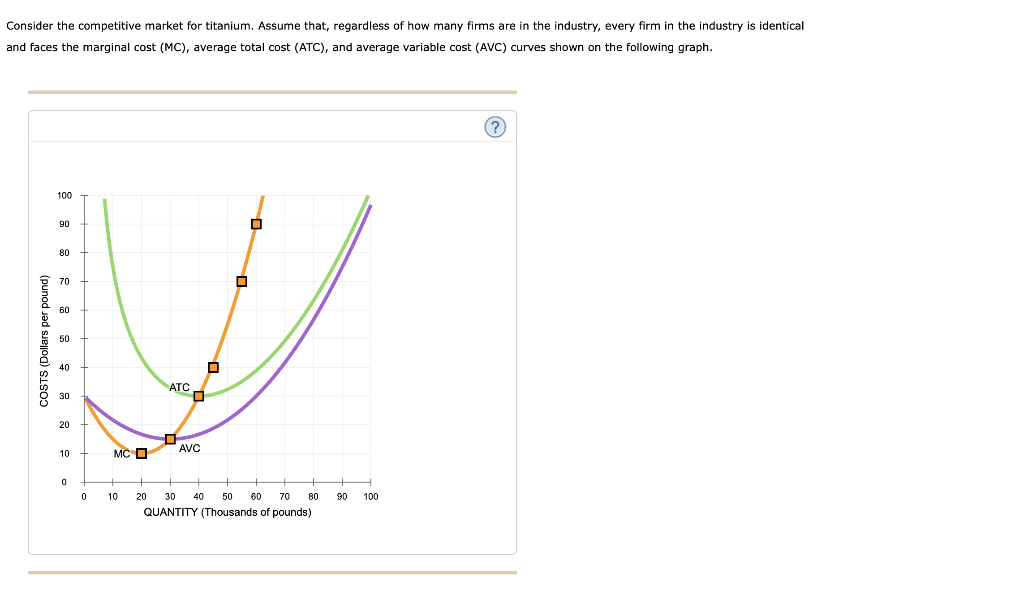5. Short-run supply and long-run equilibrium Consider the competitive market for titanium. Assume that, regardless of how many firms are in the industry, every firm in the industry is identical and faces the marginal cost (MC), average total cost (ATC), and average variable cost (AVC) curves shown on the following graph. Consider the competitive market for titanium. Assume that, regardless of how many firms are in the industry, every firm in the industry is identical and faces the marginal cost...

• ### 7. Short-run supply and long-run equilibrium Consider the competitive market for copper. Assume that, regardless of...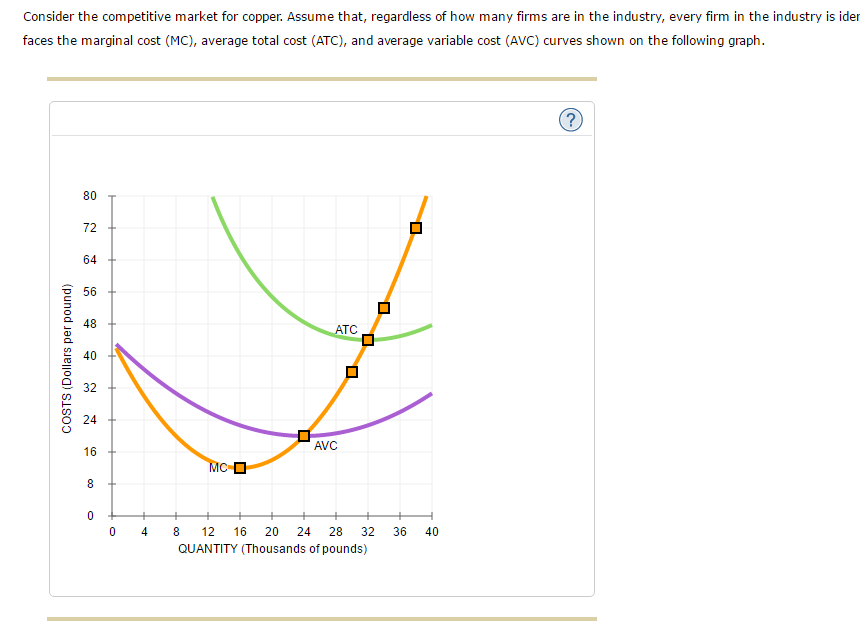7. Short-run supply and long-run equilibrium Consider the competitive market for copper. Assume that, regardless of how many firms are in the industry, every firm in the industry is identical and faces the marginal cost (MC), average total cost (ATC), and average variable cost (AVC) curves shown on the following graph. The following diagram shows the market demand for copper. Use the orange points (square symbol) to plot the initial short-run industry supply curve when there are 10 firms in...

• ### 7. Short-run supply and long-run equilibrium Consider the competitive market for titanium. Assume that, regardless of...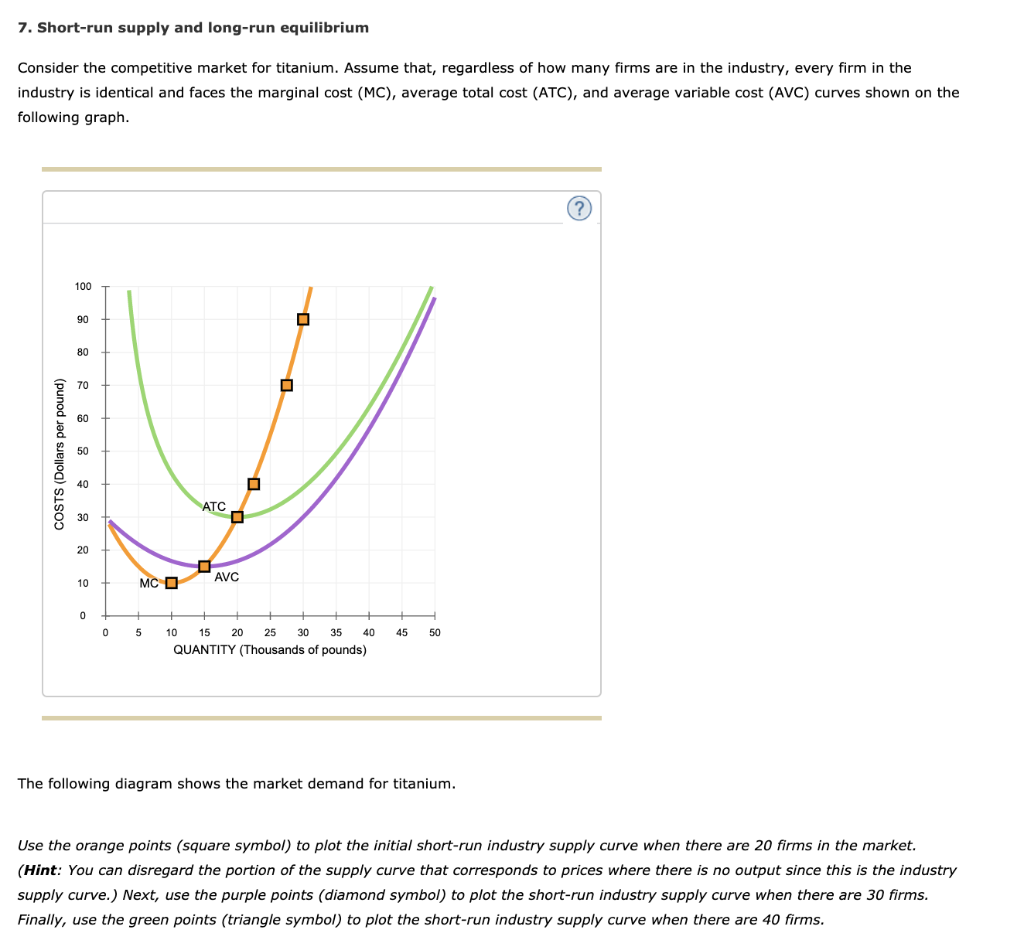7. Short-run supply and long-run equilibrium Consider the competitive market for titanium. Assume that, regardless of how many firms are in the industry, every firm in the industry is identical and faces the marginal cost (MC), average total cost (ATC), and average variable cost (AVC) curves shown on the following graph. COSTS (Dollars per pound) + MC O AVC 0 5 45 50 10 15 20 25 30 35 40 QUANTITY (Thousands of pounds) The following diagram shows the market...

Need Online Homework Help?